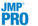Publication date: 07/30/2020

##Variogram

A Variogram plot describes the spatial or temporal correlation of observations in terms of their distance. The plot shows the semivariance as a function of distance or time. The theoretical semivariance is one half of the variance of the difference between response values at locations that are a given distance apart. Note that semivariance and correlation at a given distance are inversely related. If the correlation between values at a given distance is small, the semivariance between observations at that distance is large.

When you specify any isotropic Repeated Structure (AR(1), Spatial, or Spatial with Nugget) in the Fit Model window, a Variogram plot is shown by default. If you specify the Residual structure, selecting the Variogram option in the red triangle menu enables you to select the continuous columns to be used in calculating the variogram. You can include any number of columns that describe the spatial or temporal structure of your data.

The initial Variogram report shows a plot of the empirical semivariance against distance. For additional background and more information, see Antedependent Covariance Structure.

#### Semivariance Curves for Isotropic Structures

Semivariance curves are provided for the isotropic covariance structures: AR(1), Power, Exponential, Gaussian, and Spherical. For the spatial structures, curves are provided for models with and without nuggets.

The curves for the theoretical models are fit using the covariance parameter estimates. For the underlying formulas, see Chilès and Delfiner (2012) and Cressie (1993).

Use the theoretical models to determine whether your data conform to your selected isotropic structure. If you have selected the Residual structure, you can use the empirical variogram to determine whether your data exhibit some temporal or spatial structure. If the points seem follow a horizontal line, this suggests that the correlation does not change with distance and that the Residual structure is appropriate. If the points show a pattern, fitting various isotropic models might suggest an appropriate Repeated structure with which to refit your model.

###Nugget

The nugget is the vertical jump from the value of 0 at the origin of the variogram to the value of the semivariance at a very small separation distance. A variogram model with a nugget has a discontinuity at the origin. The value of the theoretical curve for distances just above 0 is the nugget.

###Variogram Options

AR(1)

Plots a variogram for an AR(1) covariance structure.

Spatial

Plots a variogram for an Exponential, Gaussian, Power, or Spherical covariance structure.

Spatial with Nugget

Plots a variogram for an Exponential, Gaussian, Power, or Spherical covariance structure with nugget.